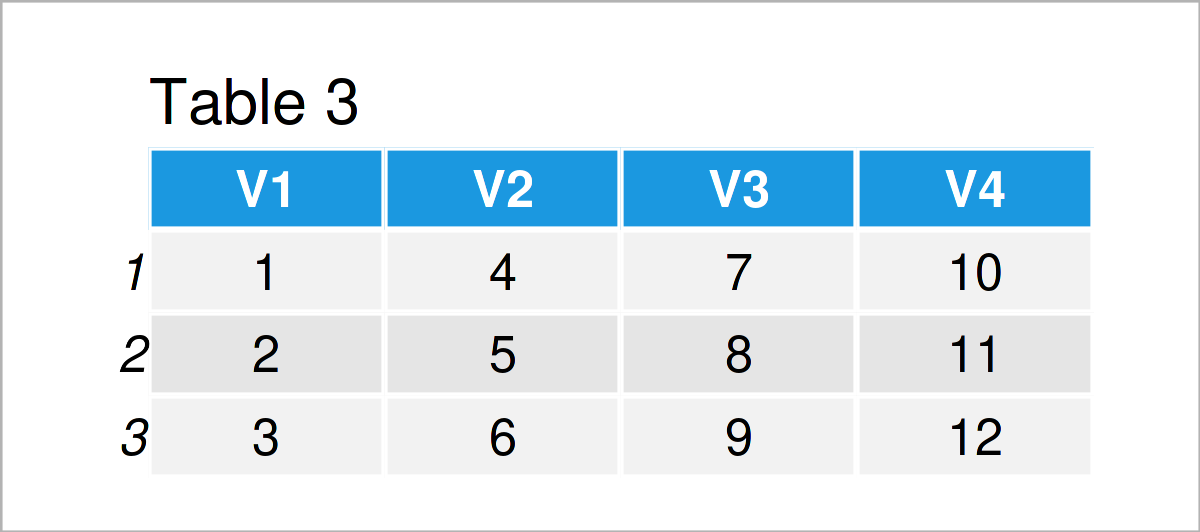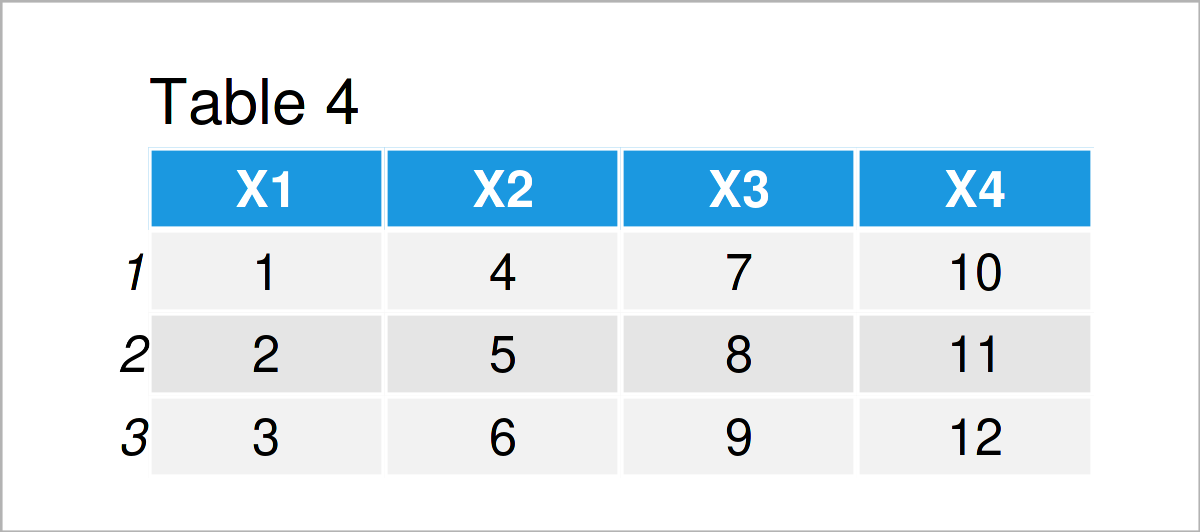# Difference Between data.frame & as.data.frame Functions in R (2 Examples)

In this post, I’ll demonstrate how to apply the data.frame and as.data.frame functions in the R programming language.

Let’s just jump right in:

## Example 1: Create Data Frame Using data.frame() Function

In Example 1, I’ll demonstrate how to use the data.frame function in R. Usually, the data.frame function is used to construct a new data object with the data.frame class.

Have a look at the R code below. In this syntax, we specify several columns (i.e. x1, x2, and x3) within the data.frame function. Furthermore, we are storing the output of the data.frame function in a new data object called data1.

```data1 <- data.frame(x1 = 1:5,    # Create data frame
x2 = 2:6,
x3 = letters[10:6])
data1                            # Print data frame```The output of the previous syntax is shown in Table 1 – A new data frame containing three variables with different values.

## Example 2: Convert Matrix to Data Frame Using as.data.frame() & data.frame() Functions

In Example 2, I’ll demonstrate how to apply the as.data.frame function. The as.data.frame function is typically used to coerce data sets with other classes to the data.frame class.

Let’s first create a matrix object:

```mat <- matrix(1:12, ncol = 4)    # Create matrix
mat                              # Print matrix```In Table 2 it is shown that we have created a matrix containing three rows and four columns.

Now, we can use the as.data.frame function to convert this matrix to the data.frame class:

```data2 <- as.data.frame(mat)      # Convert matrix to data frame
data2                            # Print data frame```By executing the previous R code, we have created Table 3, i.e. a data frame containing the values of our input matrix.

Note that we could also use the data.frame function to convert a matrix to a data.frame (even though this is not the main purpose of the data.frame function):

```data3 <- data.frame(mat)         # Convert matrix to data frame
data3                            # Print data frame```The output of the previous R code is shown in Table 4 – Another data frame with the same values. Note that the variable names of this data frame are different compared to the data frame created by the as.data.frame function.

## Video, Further Resources & Summary

Some time ago, I have published a video on my YouTube channel, which illustrates the R programming code of this post. Please find the video below:

Please accept YouTube cookies to play this video. By accepting you will be accessing content from YouTube, a service provided by an external third party.If you accept this notice, your choice will be saved and the page will refresh.

Besides that, you might read some of the related articles on this homepage: